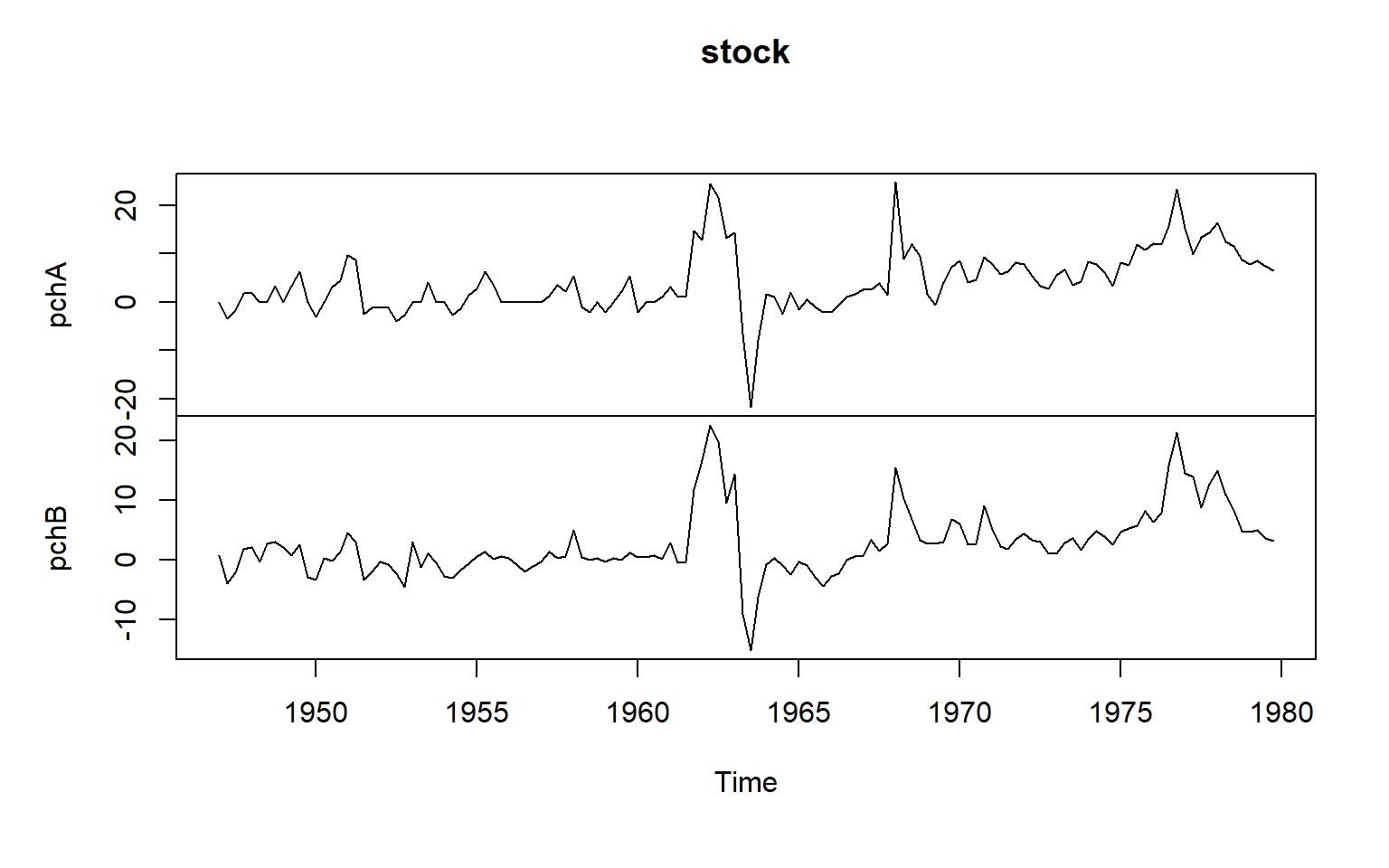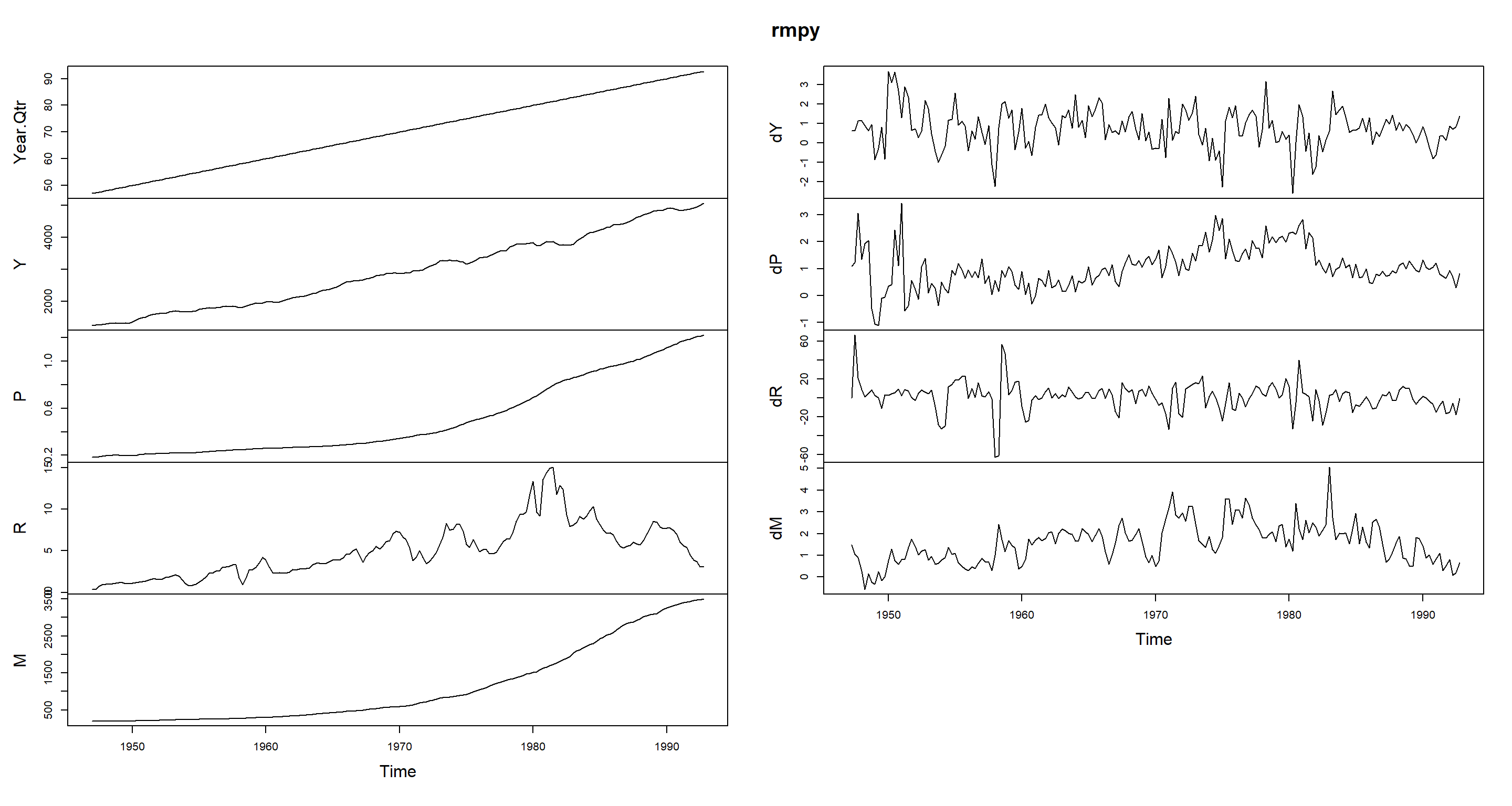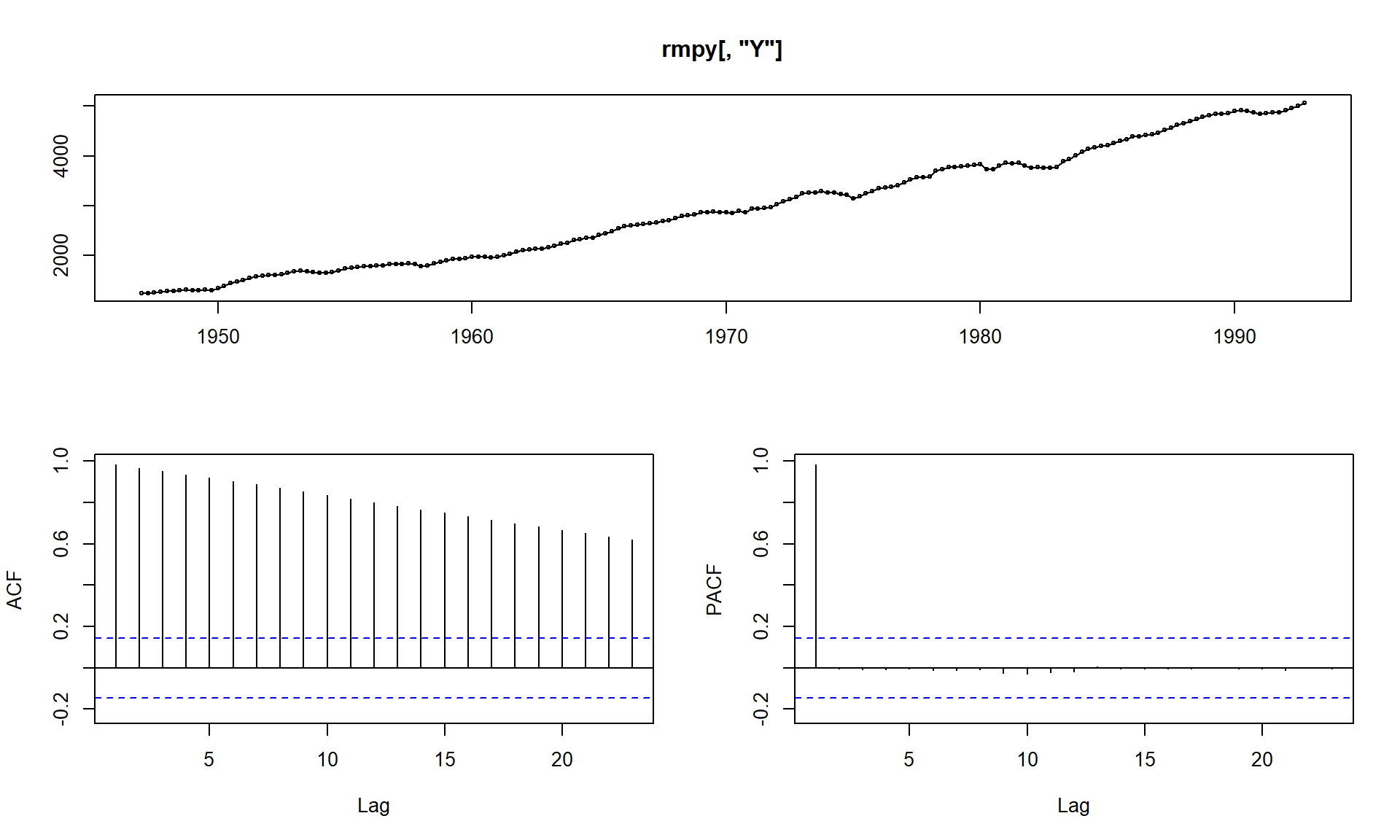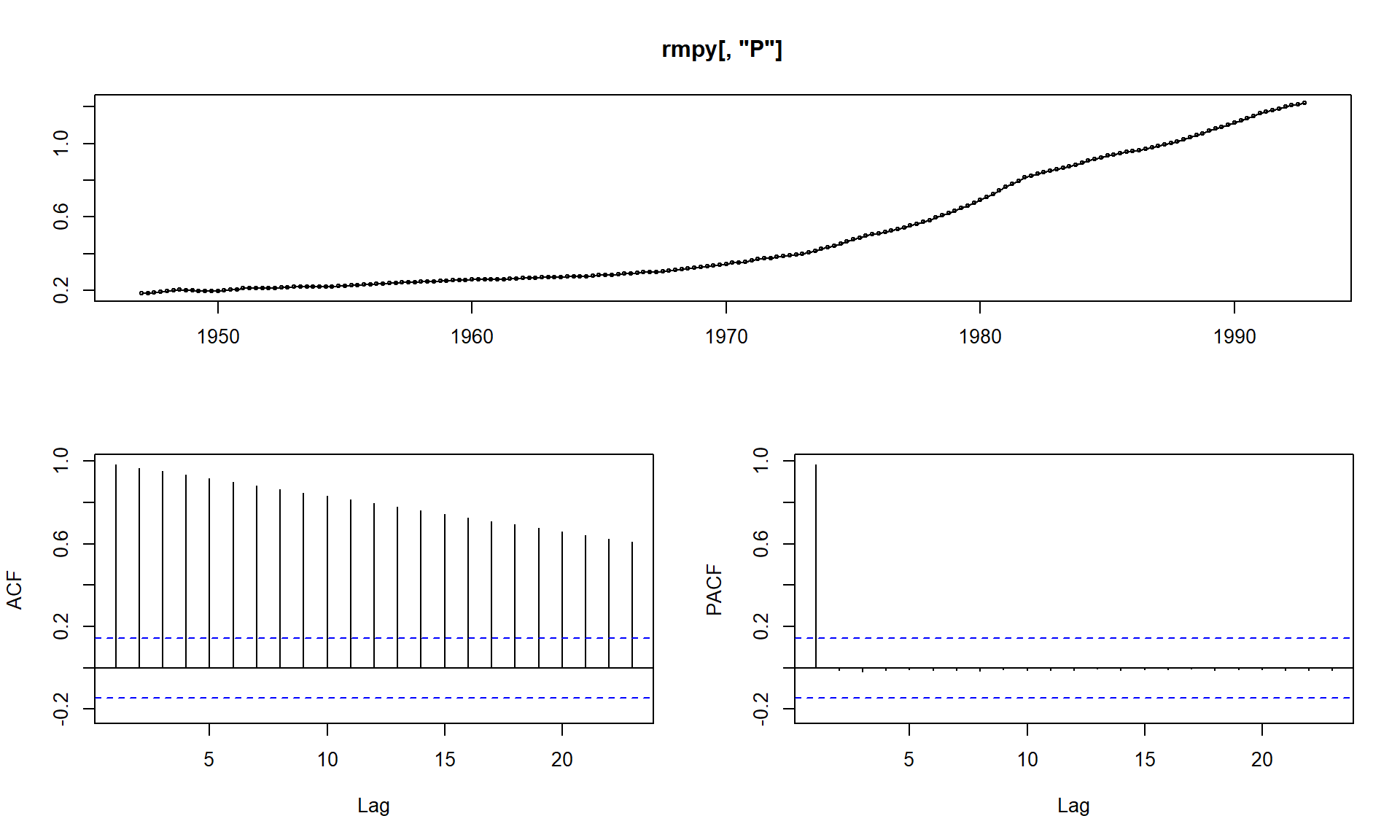# Granger Causality

The file stockpab.xls contains 133 monthly data of the most important stock price indices in Countries A and B (the data are logged) and respective stock market returns:

• pchA stock returns in Country A
• pchB stock returns in Country B

Lets assume that both indices have unit roots but are not cointegrated. On the other hand, the logarithmic differences, i.e., returns, are stationary. We are interested in whether the stock returns of country A Granger causes the returns in B.

suppressPackageStartupMessages({
suppressWarnings({
library(dynlm)
library(forecast)
library(urca)
library(vars)
})
})
txt1 <- "http://uosis.mif.vu.lt/~rlapinskas/(data%20R&GRETL/"
txt2 <- "stockpab.xls"
tmp = tempfile(fileext = ".xls")
stock <- ts(stock[-1, 3:4], frequency = 4, start = c(1947, 1))
plot.ts(stock)We have already learned of the sequential procedure to choose p and q in the model. On the other hand, in the Granger causality case, this is not the question of the first importance (and, also, we usually assume p = q ).

## The restricted model:

We will begin by estimating the Restricted model: $Y_t = \alpha + \delta t + \phi Y_{t-1} + ... + \phi_p Y_{t-p} + \epsilon_t$

mod1R=dynlm(pchA~time(stock)+L(pchA,1:2),data=stock)
summary(mod1R)
##
## Time series regression with "ts" data:
## Start = 1947(3), End = 1979(4)
##
## Call:
## dynlm(formula = pchA ~ time(stock) + L(pchA, 1:2), data = stock)
##
## Residuals:
##      Min       1Q   Median       3Q      Max
## -18.1491  -1.9509  -0.3877   1.8186  21.6793
##
## Coefficients:
##                 Estimate Std. Error t value        Pr(>|t|)
## (Intercept)   -273.12037   99.28645  -2.751         0.00682 **
## time(stock)      0.14002    0.05065   2.764         0.00656 **
## L(pchA, 1:2)1    0.65583    0.08872   7.392 0.0000000000176 ***
## L(pchA, 1:2)2   -0.07830    0.08876  -0.882         0.37936
## ---
## Signif. codes:  0 '***' 0.001 '**' 0.01 '*' 0.05 '.' 0.1 ' ' 1
##
## Residual standard error: 4.547 on 126 degrees of freedom
## Multiple R-squared:  0.5257, Adjusted R-squared:  0.5144
## F-statistic: 46.55 on 3 and 126 DF,  p-value: < 0.00000000000000022

## The unrestricted model

Now we estimate the unrestricted model: $Y_t = \alpha + \delta t + \phi_1 Y_{t-1} + ... + \phi_p Y_{t-p} + \beta_1 X_{t-1} + ... + \beta_q X_{t-q} + \epsilon_t$

mod1NR=dynlm(pchA~time(stock)+L(pchA,1:2)+L(pchB,1:2),data=stock)
summary(mod1NR)
##
## Time series regression with "ts" data:
## Start = 1947(3), End = 1979(4)
##
## Call:
## dynlm(formula = pchA ~ time(stock) + L(pchA, 1:2) + L(pchB, 1:2),
##     data = stock)
##
## Residuals:
##      Min       1Q   Median       3Q      Max
## -19.7168  -2.1826  -0.2584   1.9523  19.7466
##
## Coefficients:
##                   Estimate   Std. Error t value  Pr(>|t|)
## (Intercept)   -290.8565848   93.6062093  -3.107   0.00234 **
## time(stock)      0.1492898    0.0477631   3.126   0.00221 **
## L(pchA, 1:2)1    0.0489767    0.1659134   0.295   0.76834
## L(pchA, 1:2)2   -0.0007376    0.1656757  -0.004   0.99646
## L(pchB, 1:2)1    0.8607950    0.1977275   4.353 0.0000277 ***
## L(pchB, 1:2)2   -0.2339125    0.2026224  -1.154   0.25055
## ---
## Signif. codes:  0 '***' 0.001 '**' 0.01 '*' 0.05 '.' 0.1 ' ' 1
##
## Residual standard error: 4.268 on 124 degrees of freedom
## Multiple R-squared:  0.5887, Adjusted R-squared:  0.5721
## F-statistic:  35.5 on 5 and 124 DF,  p-value: < 0.00000000000000022

## Model Comparison

To compare these two models by their SSR, we can use the F statistics and anova function:

anova(mod1NR, mod1R)
## Analysis of Variance Table
##
## Model 1: pchA ~ time(stock) + L(pchA, 1:2) + L(pchB, 1:2)
## Model 2: pchA ~ time(stock) + L(pchA, 1:2)
##   Res.Df    RSS Df Sum of Sq      F    Pr(>F)
## 1    124 2258.8
## 2    126 2604.9 -2   -346.13 9.5005 0.0001449 ***
## ---
## Signif. codes:  0 '***' 0.001 '**' 0.01 '*' 0.05 '.' 0.1 ' ' 1

With the p-value = 0.0001449 < 0.05, we reject the null hypothesis that the models are identical, thus the information on pchB notably improves the forecast of pchA. On the other hand, on reversing the procedure, we can see that pchA is not the Granger cause of pchB:

mod2NR=dynlm(pchB~time(stock)+L(pchB,1:2)+L(pchA,1:2),data=stock)
summary(mod2NR)
##
## Time series regression with "ts" data:
## Start = 1947(3), End = 1979(4)
##
## Call:
## dynlm(formula = pchB ~ time(stock) + L(pchB, 1:2) + L(pchA, 1:2),
##     data = stock)
##
## Residuals:
##      Min       1Q   Median       3Q      Max
## -19.8898  -1.5177  -0.2520   0.8356  12.1289
##
## Coefficients:
##                 Estimate Std. Error t value   Pr(>|t|)
## (Intercept)   -185.63874   78.88663  -2.353     0.0202 *
## time(stock)      0.09513    0.04025   2.363     0.0197 *
## L(pchB, 1:2)1    0.82706    0.16663   4.963 0.00000224 ***
## L(pchB, 1:2)2   -0.05817    0.17076  -0.341     0.7339
## L(pchA, 1:2)1   -0.01806    0.13982  -0.129     0.8975
## L(pchA, 1:2)2   -0.09376    0.13962  -0.672     0.5031
## ---
## Signif. codes:  0 '***' 0.001 '**' 0.01 '*' 0.05 '.' 0.1 ' ' 1
##
## Residual standard error: 3.597 on 124 degrees of freedom
## Multiple R-squared:  0.6066, Adjusted R-squared:  0.5907
## F-statistic: 38.24 on 5 and 124 DF,  p-value: < 0.00000000000000022
mod2R=dynlm(pchB~time(stock)+L(pchB,1:2),data=stock)
summary(mod2R)
##
## Time series regression with "ts" data:
## Start = 1947(3), End = 1979(4)
##
## Call:
## dynlm(formula = pchB ~ time(stock) + L(pchB, 1:2), data = stock)
##
## Residuals:
##      Min       1Q   Median       3Q      Max
## -20.0611  -1.6162  -0.3550   0.8346  12.1037
##
## Coefficients:
##                 Estimate Std. Error t value             Pr(>|t|)
## (Intercept)   -173.19420   75.63878  -2.290               0.0237 *
## time(stock)      0.08872    0.03857   2.300               0.0231 *
## L(pchB, 1:2)1    0.80997    0.08760   9.246 0.000000000000000746 ***
## L(pchB, 1:2)2   -0.15727    0.08770  -1.793               0.0753 .
## ---
## Signif. codes:  0 '***' 0.001 '**' 0.01 '*' 0.05 '.' 0.1 ' ' 1
##
## Residual standard error: 3.575 on 126 degrees of freedom
## Multiple R-squared:  0.605,  Adjusted R-squared:  0.5956
## F-statistic: 64.34 on 3 and 126 DF,  p-value: < 0.00000000000000022
anova(mod2NR, mod2R)
## Analysis of Variance Table
##
## Model 1: pchB ~ time(stock) + L(pchB, 1:2) + L(pchA, 1:2)
## Model 2: pchB ~ time(stock) + L(pchB, 1:2)
##   Res.Df    RSS Df Sum of Sq      F Pr(>F)
## 1    124 1604.3
## 2    126 1610.5 -2   -6.2165 0.2402 0.7868

with p-value = 0.7868 > 0.05 we do not reject the null hypothesis that $$SSR_{UR} \sim SSR_R$$.

Note that we can shorten the whole procedure with:

#'both' means that the model includes both a constant and a trend
var.2c <- VAR(stock, p=2, type="both")
causality(var.2c, cause = "pchA")
## $Granger ## ## Granger causality H0: pchA do not Granger-cause pchB ## ## data: VAR object var.2c ## F-Test = 0.24025, df1 = 2, df2 = 248, p-value = 0.7866 ## ## ##$Instant
##
##  H0: No instantaneous causality between: pchA and pchB
##
## data:  VAR object var.2c
## Chi-squared = 54.14, df = 1, p-value = 0.0000000000001866
causality(var.2c, cause = "pchB")
## $Granger ## ## Granger causality H0: pchB do not Granger-cause pchA ## ## data: VAR object var.2c ## F-Test = 9.5005, df1 = 2, df2 = 248, p-value = 0.0001058 ## ## ##$Instant
##
##  H0: No instantaneous causality between: pchB and pchA
##
## data:  VAR object var.2c
## Chi-squared = 54.14, df = 1, p-value = 0.0000000000001866

with p-value = 0.7866 we do not reject Granger causality H0: pchA do not Granger-cause pchB. with p-value = 0.0001058 we reject Granger causality H0: pchB do not Granger-cause pchA.

# VAR: Estimation and Forecasting

Economists often use such important macroeconomic variables as: R = the interest rate, M = the money supply, P = the price level and Y = real GDP. Due to the symbols used, models using these variables are sometimes informally referred to as RMPY models

The file rmpy.xls contains quarterly data on the variables for the US from 1947:1 through 1992:4. To be precise:

• r three-month Treasury bill rate
• m money supply (M2) measured in billions of dollars
• p price level measured by the GDP deflator (a price index with 1987 = 1.00)
• y GDP measured in billions of 1987 dollars

Before carrying out an analysis using time series data, you must conduct unit root tests. Remember that, if unit roots are present but cointegration does not occur, then the spurious regression problem exists. In this case, you should work with differenced data. Alternatively, if unit roots exist and cointegration does occur, then you will have important economic information that the series are trending together and use ECM.

suppressPackageStartupMessages({
library(dynlm)
library(forecast)
library(urca)
library(vars)
})
txt1 <- "http://uosis.mif.vu.lt/~rlapinskas/(data%20R&GRETL/"
txt2 <- "RMPY.xls"
tmp = tempfile(fileext = ".xls")
rmpy <- ts(rmpy, frequency = 4, start = c(1947, 1))
plot.ts(rmpy)tsdisplay(rmpy[, "Y"])tsdisplay(rmpy[, "P"])tsdisplay(rmpy[, "R"])